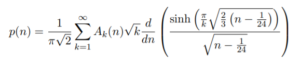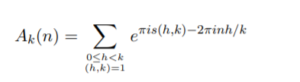# Partition Function P, Q: Simple Definition, Examples

## Partition Function in Mathematics

There are two partition functions in math:

1. P (unrestricted)
2. Q (restricted).

## 1. P (Unrestricted)

P tells you how many ways you can write an integer as a sum of positive integers. For example, you can write the number 5 as:

• 5
• 4 + 1
• 3 + 1 + 1
• 3 + 2
• 2 + 2 + 1
• 2 + 1 + 1 + 1
• 1 + 1 + 1 + 1 + 1

The number of partitions in the above example is written as P(5) = 7·(n).

Which order the summands (i.e. the quantities being added) are written in doesn’t matter. For example, 3 + 2 is the same as 2 + 3. However, the partitions are traditionally ordered from largest to smallest (Skiena, 1990, p. 51).

You can find a list of numbers and their partitions at the Online Encyclopedia of Integer Sequences. The first dozen (for integers 0 through 12) are:
1, 1, 2, 3, 5, 7, 11, 15, 22, 30, 42, 56.

An exact formula for p(n) is given by Rademacher’s convergent series formula, which surprisingly (given its complexity!) gives p(n) for any integer greater than or equal to 1:Where:and s(h, k) is a Dedekind sum:## 2. Q (Restricted)

The partition function Q is very similar, except that the numbers in each partition have to be unique. For example, the partition {2 + 2 + 1} in the above example would not count. In fact, the only partitions that would count are:

• 5
• 4 + 1
• 3 + 2

OEIS also has a list of the number of distinct partitions, the first dozen of which are: 1, 1, 1, 2, 2, 3, 4, 5, 6, 8, 10, 12.

## Partition Function in Statistical Mechanics

Every significant macroscopic quantity in a system can be expressed by a partition function. It’s a measure of how particles are spread out (i.e. partitioned among) energy levels in a system.

The partition function can be simply stated as the following ratio:

Q = N / N0

Where:

• N = the total number of particles in a system,
• N0 = the number of particles in the ground state.

The value for the ratio varies from 1 (the lowest value, when the temperature of the system is 0 degrees K) to extremely high values for very high temperature and where the spacing between every levels is tiny.

## The Boltzmann Population Formula

The partition function has its origins in the Boltzmann Population formula, found in statistical mechanics:

Q = Σj ωj exp (-Ej /kT

can be found as the denominator in the Boltzmann population formula, which expresses the probability of finding a system in a particular quantum state, j:

Pj = ωj exp(- Ej / kT) / Q

Where:

• Ej = energy of the quantum state,
• T = temperature in K,
• ωj = degeneracy of the jth state,
• Q = the partition function.

The partition function can be written in various other ways, such as the sum of terms seen here:## References

Apostol, T. M. “Rademacher’s Series for the Partition Function.” Ch. 5 in Modular Functions and Dirichlet Series in Number Theory, 2nd ed. New York: Springer-Verlag, pp. 94-112, 1997.
Ault, A. (2008). The Partition Function: If That’s What It Is Why Don’t They Say So!
December 23, 2019 from: https://people.cornellcollege.edu/aault/Chemistry/PartitionFunction1.pdf
Calkin et. al. (2006). Computing the integer partition function. Retrieved December 23, 2019 from: http://www.math.clemson.edu/~kevja/PAPERS/ComputingPartitions-MathComp.pdf
Oldham, K. et al. (2010). An Atlas of Functions. Springer.
Chapter 7: Statistical Mechanics.
Skiena, S. Implementing Discrete Mathematics: Combinatorics and Graph Theory with Mathematica. Reading, MA: Addison-Wesley, 1990.

CITE THIS AS:
Stephanie Glen. "Partition Function P, Q: Simple Definition, Examples" From StatisticsHowTo.com: Elementary Statistics for the rest of us! https://www.statisticshowto.com/partition-function/